Online Tution   »   Education News   »   CBSE Class 12 Computer Science Answer...

# Class 12 Computer Science Answer Key 2023, For Set 1,2,3 Paper PDF

## Class 12 Computer Science Answer Key 2023

CBSE Class 12 Computer Science Answer Key 2023: Central Board of Secondary Education (CBSE) is conducting the Class 12th Computer Science exam on 23 March 2023 across the country. After the test is over, students can download the Class 12 Computer Science Answer Key 2023 from this page. The Class 12 Computer Science exam will take place between 10:30 am and 01:30 pm. In this article, we have provided the CBSE Class 12 Computer Science Answer Key . The CBSE Class 12 Computer Science Answer Key is prepared by our expert faculty so students can trust this answer key and can calculate their marks.## Class 12 Computer Answer Key

We have provided some basic information on CBSE Class 12 Computer Science Answer Key. Students who took the class 12 Computer Science examination should go over the details in the table below:

 CBSE Class 12 Computer Science Answer Key Exam Conducting Body Central Board of Secondary Education Exam & Subject Name CBSE Class 12 Computer Science Category Answer Key Exam Date 23 March 2023 Unofficial Answer Key 23 March 2023 Official Answer Key To be notified Official Website https://www.cbse.nic.in/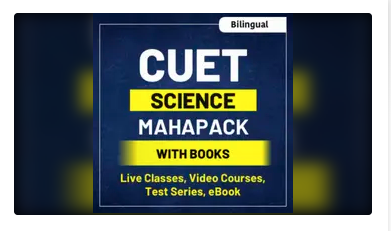## Class 12 Computer Science Answer Key & Paper Analysis

We spoke with students who took the class 12 computer science test, and their initial opinion was that the CBSE class 12 Computer Science Question paper was of moderate difficulty. A few candidates thought the exam was difficult. Section A of the exam, which consisted of brief objective questions, was the easiest and most scoring segment. The programming problems are harder than the objective theoretical questions. The questions were straightforward and followed the structure of the sample papers. The entire CBSE class 12 question paper analysis will be provided soon. Keep tuned.

CUET 2023 (प्रतिज्ञा Batch) For Science Domain Subjects Online Live Classes

### Class 12 Computer Science Answer key & Analysis 2023

 CBSE Class 12 Computer Science Answer key  & Analysis 2023 Sections Difficulty level Section A(MCQs) Easy Section B Easy Section C Easy to Moderate Section D Easy to Moderate Section E Moderate Overall difficulty level Easy to Moderate

## Class 12 Computer Science Answer Key Set 4

Here we have provided the Option Numbers of Class 12 Computer Science Answer Key 2023 Set 1. Candidates who got the Class 12 Computer Science Set 4 Question Paper can cross-check their Answers with the Class 12 Computer Science Answer Key 2023 Set 4

 Section A (Code 91) (1) True (7) (a) sort () (13) (c) MICROWAVES (2) (b) return (8) (b) (14) (c) 10, 2 (3) (b) Tup.insert (2, 3) (9) (d) Statements 2 and 4 (15) (a) count () (4) (a) True (10) (d) Cardinality (16) (b) List of tuples (5) (b) ( ‘ Amrit ‘, ‘ Mahotsav ‘ , ‘ @ ‘, ‘ 75’ ) (11) (a) 0 (17) To be Updated (6) (d) a (12) (b) ORDER BY (18) To be Updated

### CBSE Class 12 Computer Science Answer Key 2023 Set 4

1. State True or False.

“Identifiers are names used to identify a variable, function in a program”.

2. Which of the following is a valid keyword in Python?

(a) false

(b) return

(c) non local

(d) none

3. Given the following Tuple Tup- (10, 20, 30, 50)

Which of the following statements will result in an error?

(a) print (Tup)

(b) Tup.insert (2,3)

(c) print (Tup[1:2])

(d) print (len (Tup))

4. Consider the given expression: 5<10 and 12>7 or not 7>4

Which of the following will be the correct output, if the given expression is evaluated?

(a) True

(b) False

(c) NONE

(d) NULL

5. Select the correct output of the code:

“Amrit Mahotsav @ 75”

A=S.partition (“  “)

print (a)

(a)(Amrit Mahotsav’, ‘@’, ’75)

(b)[‘Amrit’, ‘Mahotsav’, ‘@’, ’75]

(c) (‘Amrit’, ‘Mahotsav’ @ 75′)

(d) (‘Amrit’,’’, ‘Mahotsav @ 75’)

6. Which of the following mode keeps the file offset position at the end of the file?

(a) r+

(b) r

(c) w

(d) a

7. Fill in the blanks.

_______function is used to arrange the elements of a list in ascending order.

(a) sort()

(b) ascending ()

(c) arrange ()

(d) asort()

8. Which of the following operations will return either True or False?

(a) +=

(b) !=

(c) =

(d) *=

9. Which of the following statement(s) would give an error after executing the following code?

Stud (“Murugan”:100,”Mithu”:95) #Statement 1

print (Stud) # Statement 2

Stud [“Murugan”]=99 # Statement 3

print (Stud.pop()) # Statement 4

print (Stud) # Statement 5

(a) Statement 2

(c) Statement 4

(b) Statement 3

(d) Statements 2 and 4

Answer: (d) Statements 2 and 4

10. Fill in the blank.

_____is the number of tuples in a relation.

(a) Attribute

(b) Degree

(c) Domain

(d) Cardinality

11. The syntax of seek() is :

file_object.seek (offset [, reference_point])

What is the default value of reference_point?

(a) 0

(b) 1

(c) 2

(d) 3

12. Fill in the blank:

_______clause is used with SELECT statement to display data in a sorted form with respect to a specified column.

(a) WHERE

(b) ORDER BY

(c) HAVING

(d) DISTINCT

13. Fill in the blank:

__________is used for point-to-point communication or unicast communication such as radar and satellite.

(a) INFRARED WAVES

(c) MICROWAVES

(b) BLUETOOTH

14. What will the following expression be evaluated to in Python?

/print (4+3 5/3-5%2)

(a) 8.5

(b) 8.0

(c) 10.2

(d) 10.0

15. Which function returns the sum of all elements of a list?

(a) count()

(b) sum ()

(c) total()

16. fetchall0 method fetches all rows in a result set and returns a:

(a) Tuple of lists

(c) List of strings

(b) List of tuples

(d) Tuple of strings

Q. 17 and 18 are ASSERTION (A) and REASONING (R) based questions. Mark the correct choice as

(a) Both (A) and (R) are true and (R) is the correct explanation for (A).

(b) Both (A) and (R) are true and (R) is not the correct explanation for (A).

(c) (A) is true but (R) is false.

(d) (A) is false but (R) is true.

## Class 12 Computer Science Answer Key 2023 Exam Pattern

Candidate must go through the CBSE Class 12 Exam pattern and marking Scheme while analyzing the CBSE Class 12 Computer Science Answer Key 2023 given on this page. This will help you to calculate your expected score in the CBSE Class 12 Computer Science Exam. CBSE Class 12 Computer Science Question Paper 2023 The question paper is divided into five sections, Section A through Section E, totaling 70 marks. Candidates must complete the paper in three hours.

1. Section A contains 18 questions for one point each.
2. Section B contains 07 Very Short Answer type questions worth 02 points each.
3.  Section C contains 05 Short Answer questions for 3 marks each.
4. Section D contains three Long Answer style questions, each worth 05 points.
5. Section E contains two questions, each worth four points. In Q35, there is only one internal choice against Part C.

In the below section, we will provide the CBSE Class 12 Computer Science Answer Key. The CBSE Class 12 Computer Science Answer Key will be available within 1 hour after completion of the examination.

## CBSE Class 12th Computer Science Answer Key and Paper Solution

After the exam is over, students should review the correct CBSE Class 12th Computer Science Answer Key to each question on the CBSE class 12 computer science question paper. The Adda247 Experts continuously update the questions and answers for the CBSE class 12 Computer Science Test. You can use the CBSE Class 12 Computer Science Answer Key 2023 to check that all of the correct answers were given once the test is done.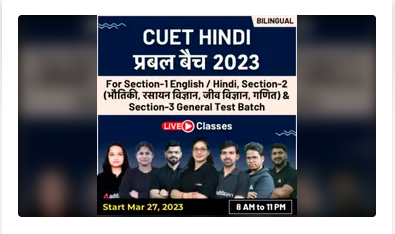## Computer Science Class 12 Answer Key 2023

Students taking the 12th Computer Science test might use their answer keys to forecast their final marks. Students will be able to access the CBSE Class 12 Computer Science Answer Key 2023 on this page. Adda247 Experts are constantly revising the CBSE class 12 Computer Science Test questions and answers. After the exam, use the CBSE Class 12 Computer Science Answer Key 2023 to ensure that all of the correct answers were provided.

## Class 12 Computer Science Answer Key- Question Paper

With the help of the Class 12 Computer Science Answer Key and the Class 12 Computer Science Question Papers, candidates can estimate their predicted results. The grading and numbers in the exam questions are the same in each set, but the questions are arranged in different numbers. In this section, we will provide the CBSE Class 12 Computer Science Question Papers 2023 Pdf for all four sets.

 CBSE Class 12 Computer Science Question Papers 2023 Pdf Download CBSE Class 12 Computer Science Question Paper 2023 Set 1 CBSE Class 12 Computer Science Question Papers 2023- Set 2 CBSE Class 12 Computer Science Question Papers 2023- Set 3

## CBSE Class 12 Computer Science Answer Key – Paper Solution

After the exam, candidates rush to find the Class 12 Computer Science Answer key 2023. We will provide the Class 12 Computer Science Answer key 2023 for all sets and Exam analysis for your convenience. We will provide the detailed 100% correct CBSE Class 12 Computer Science Answer key 2023 with question paper analysis as soon as the CBSE Class 12 Computer Science Exam 2023 is completed.

Related Post: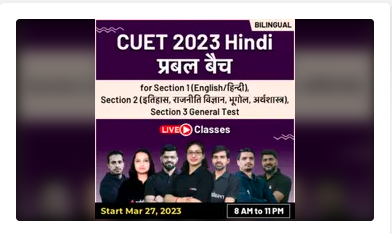Sharing is caring!

## FAQs

### Q. Where can I get Computer Science Class 12 Answer Key ?

We have provided the  Class 12 Computer Science Answer Key 2023 on this page. The students can use  Class 12 Computer Science Answer Key 2023 to calculate the marks.

### Q. Will CBSE release the official Computer Science Class 12 Answer Key 2023?

If CBSE will provide any update about the official Computer Science Class 12 Answer Key 2023 . we will update the same here, bookmark this page to get all the latest updates.

### Q. When will CBSE announce the result of the Class 12 examination?

After completion of the Class 12th examination, CBSE will announce the result which is expected in the month of May 2023.

### TOPICS:

•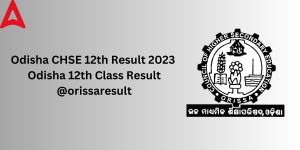CHSE Odisha Result 2023 Arts Out, 12th D...
•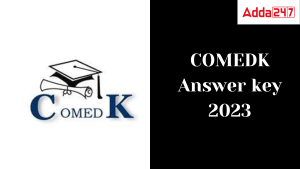COMEDK Answer key 2023 Out, Check Respon...
•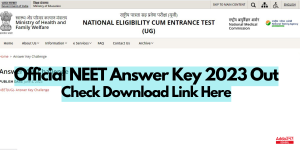NEET Answer Key 2023 Out @neet.nta.nic.i...
•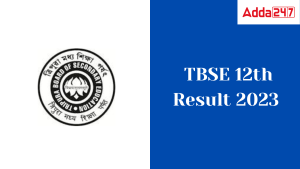TBSE Class 12 Result 2023 Out, Tripura 1...
•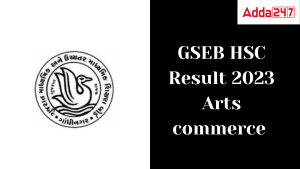GSEB HSC Result 2023 Out, Arts, Commerce...
•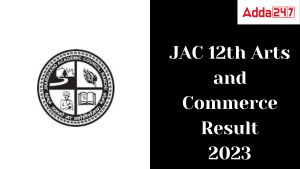JAC 12th Result 2023 Out, Arts and Comme...

Join the Conversation

1.2.1.akamia says:
2.mohtava says: